# Who I

Who I am? My third is 2 less than my half.

x =  12

### Step-by-step explanation:

x/3 = x/2-2

x = 12

Our simple equation calculator calculates it.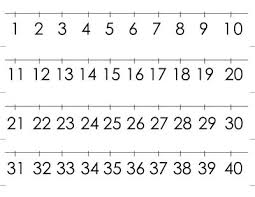Did you find an error or inaccuracy? Feel free to write us. Thank you!Tips to related online calculators
Need help to calculate sum, simplify or multiply fractions? Try our fraction calculator.
Do you have a linear equation or system of equations and looking for its solution? Or do you have a quadratic equation?

## Related math problems and questions:

• TimeageSeven times my age is 8, less than the largest two-digit number. How old am I?
• Two numbersThe sum of two numbers is 1. Identify this two numbers if you know that the half of first is equal to the third of second number.
• Half of 2Half of Ethan’s string is equal to 2/3 of Kayla’s string. The total length of their strings is 10 feet. How much longer is Ethan’s string than Kayla’s?
• Unknown number 22I am a two-digit number. My tens digits are 4. My one digit is three more than my tens. Who am I?
• Four pupilsFour pupils divided \$ 1485 so that the second received 50% less than the first, the third 1/2 less than a fourth, and fourth \$ 154 less than the first. How much money had each of them?
• Equation with xSolve the following equation: 2x- (8x + 1) - (x + 2) / 5 = 9
• Three men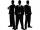Alex is half younger than Jan, which is one-third younger than George. The sum of their ages is 48. How are these three men old?
• Mr. Happy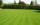Mr. Happy planted 36.6 meters square gardens grass; It's a third of the garden more than half of the garden. What is square area of this garden?
• Two numbers 11The sum of two rational numbers is (-2). If one of them is 3/5, find the other.
• What is 9What is the value of x in the proportion 2 and one-fourth over x = 1 and one-half over 3 and three-fifths? 2 and two-fifths 5 and two-fifths 8 and 1 over 10 12 and 3 over 20
• Equation with fractionsSolve an equation: 1- (2x-5)/(6) =3- (x)/(4) It is an equation with fractions.
• When I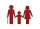When I am as old as my older brother is today, my brother will be twice as old as I am today. a) How old is my brother if I am 8 years old today? b) How old am I if my brother is 21 today?
• If-then equationIf 1/2 + 2/5s = s - 3/4 what is the value of s?
• Tape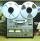Video is 153% more expensive than tape recorder. How many percent is tape recorder less expensive than video?
• Roses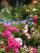On the large rosary was a third white, half red, yellow quarter and six pink. How many roses was in the rosary?
• Hockey teams goals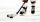Hockey teams fired 200 goals. The second team 13 less than first team, third 16 less than the first and fourth tean 19 goals less than first. How many goals fired each team?
• Class 8.AThree quarters of class 8.A went skiing. Of those who remained at home one third was ill and the remaining six were on math olympic. How many students have class 8.A?